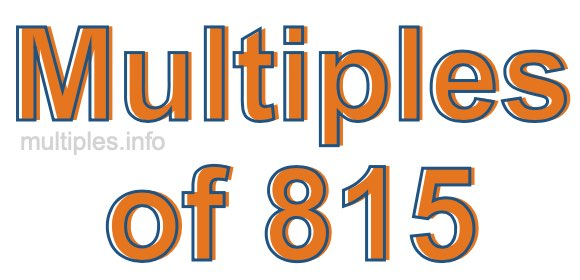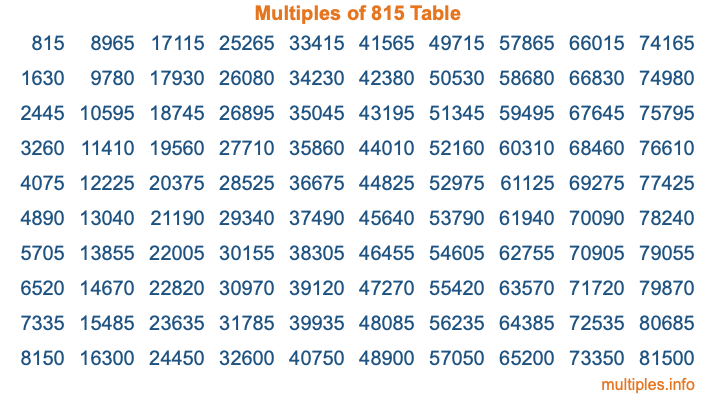Multiples of 815Welcome to the Multiples of 815 page. Here we will first teach you everything you will ever need to know about the multiples of 815, and then give you a study guide summary of everything we taught you to make sure you remember it all. Use this page to look up facts and learn information about the multiples of 815. This page will make you a multiples of eight hundred fifteen expert!

Definition of Multiples of 815
Multiples of 815 are all the numbers that when divided by 815 equal an integer. Each of the multiples of 815 are called a multiple. A multiple of 815 is created by multiplying 815 by an integer.

Therefore, to create a list of multiples of 815, you start with 1 multiplied by 815, then 2 multiplied by 815, then 3 multiplied by 815, and so on for as long as you want. Thus, the list of the first five multiples of 815 is 815, 1630, 2445, 3260, and 4075. To see a larger list of multiples of 815, see the printable image of Multiples of 815 further down on this page. We also have a category where you can choose any nth multiple of 815.

Multiples of 815 Checker
The Multiples of 815 Checker below checks to see if any number of your choice is a multiple of 815. In other words, it checks to see if there is any number (integer) that when multiplied by 815 will equal your number. To do that, we divide your number by 815. If the the quotient is an integer, then your number is a multiple of 815.

Is  a multiple of 815?

Least Common Multiple of 815 and ...
A Least Common Multiple (LCM) is the lowest multiple that two or more numbers have in common. This is also called the smallest common multiple or lowest common multiple and is useful to know when you are adding our subtracting fractions. Enter one or more numbers below (815 is already entered) to find the LCM.

Check out our LCM Calculator if you need more details about the Least Common Multiple or if you need the LCM for different numbers for adding and subtraction fractions.

nth Multiple of 815
As we stated above, 815 is the first multiple of 815, 1630 is the second multiple of 815, 2445 is the third multiple of 815, and so on. Enter a number below to find the nth multiple of 815.

th multiple of 815

Multiples of 815 vs Factors of 815
815 is a multiple of 815 and a factor of 815, but that is where the similarities end. All postive multiples of 815 are 815 or greater than 815. All positive factors of 815 are 815 or less than 815.

Below is the beginning list of multiples of 815 and the factors of 815 so you can compare:

Multiples of 815: 815, 1630, 2445, 3260, 4075, etc.

Factors of 815: 1, 5, 163, 815

As you can see, the multiples of 815 are all the numbers that you can divide by 815 to get a whole number. The factors of 815, on the other hand, are all the whole numbers that you can multiply by another whole number to get 815.

It's also interesting to note that if a number (x) is a factor of 815, then 815 will also be a multiple of that number (x).

Multiples of 815 vs Divisors of 815
The divisors of 815 are all the integers that 815 can be divided by evenly. Below is a list of the divisors of 815.

Divisors of 815: 1, 5, 163, 815

The interesting thing to note here is that if you take any multiple of 815 and divide it by a divisor of 815, you will see that the quotient is an integer.

Multiples of 815 Table
Below is an image of the first 100 multiples of 815 in a table. The table is in chronological order, column by column. The first column has the first ten multiples of 815, the second column has the next ten multiples of 815, and so on.The Multiples of 815 Table is also referred to as the 815 Times Table or Times Table of 815. You are welcome to print out our table for your studies.

Negative Multiples of 815
Although not often discussed or needed in math, it is worth mentioning that you can make a list of negative multiples of 815 by multiplying 815 by -1, then by -2, then by -3, and so on, to get the following list of negative multiples of 815:

-815, -1630, -2445, -3260, -4075, etc.

Multiples of 815 Summary
Below is a summary of important Multiples of 815 facts that we have discussed on this page. To retain the knowledge on this page, we recommend that you read through the summary and explain to yourself or a study partner why they hold true.

There are an infinite number of multiples of 815.

A multiple of 815 divided by 815 will equal a whole number.

815 divided by a factor of 815 equals a divisor of 815.

The nth multiple of 815 is n times 815.

The largest factor of 815 is equal to the first positive multiple of 815.

815 is a multiple of every factor of 815.

815 is a multiple of 815.

A multiple of 815 divided by a divisor of 815 equals an integer.

815 divided by a divisor of 815 equals a factor of 815.

Any integer times 815 will equal a multiple of 815.

Multiples of a Number
Here you can get the multiples of another number, all with the same attention to detail as we did for multiples of 815 on this page.

Multiples of
Multiples of 816
Did you find our page about multiples of eight hundred fifteen educational? Do you want more knowledge? Check out the multiples of the next number on our list!